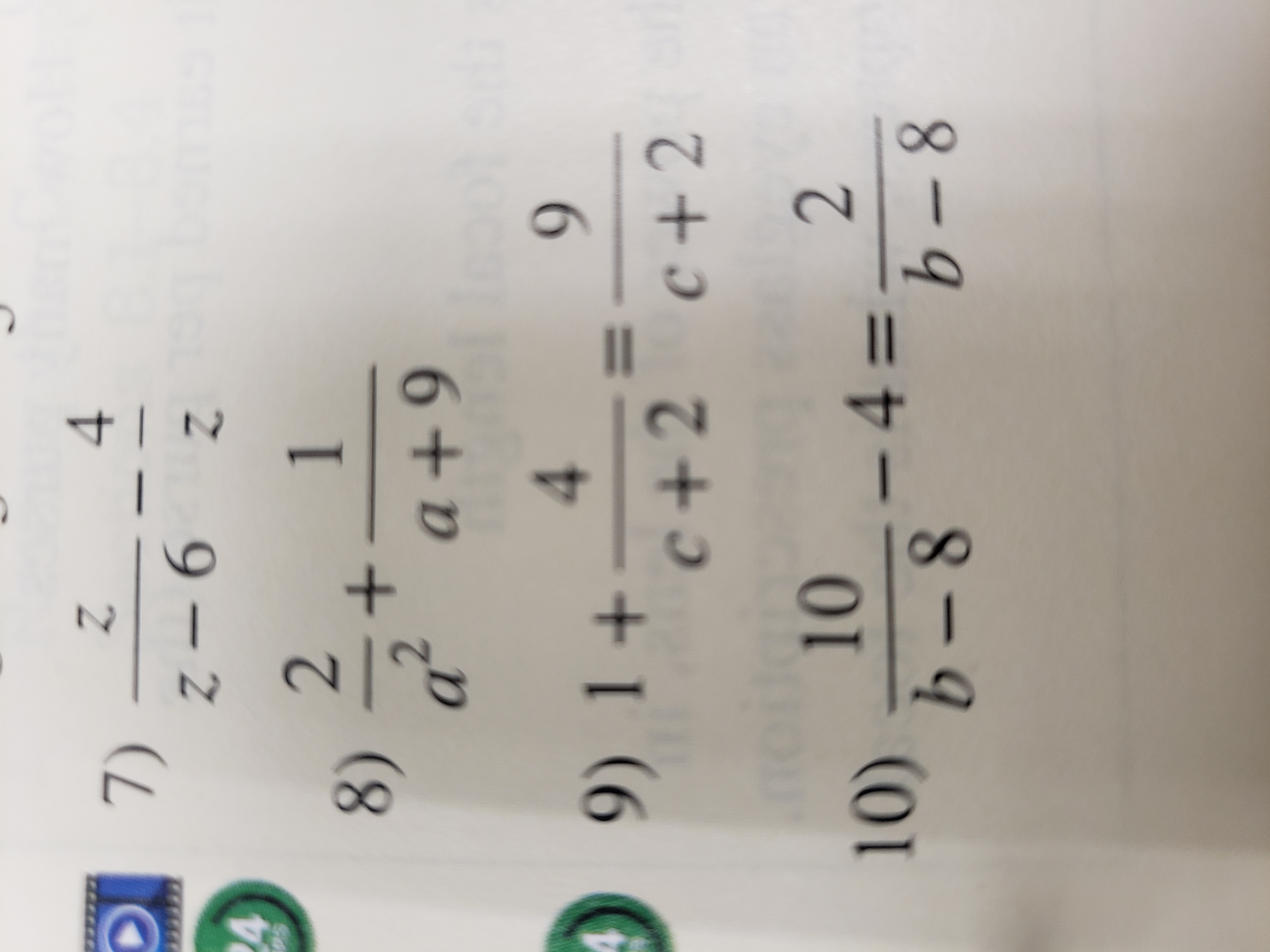# 47)Z-6421+a2a+98)a +9944 9) 1+c +2 c+2210-4=10)b-8b-8I1

Question
6 views

Solve #9

Determine whether each is an equation or is a sum of difference of expressions. Then, Solve the equation or find the sum of differencehelp_outlineImage Transcriptionclose4 7) Z-6 4 2 1 + a2a+9 8) a +9 9 4 4 9) 1+ c +2 c+2 2 10 -4= 10) b-8 b-8 I1 fullscreen
check_circle

Step 1

To solve the given equation.

Step 2

Given information:

Step 3

Calculation:

simplify the equa...

### Want to see the full answer?

See Solution

#### Want to see this answer and more?

Solutions are written by subject experts who are available 24/7. Questions are typically answered within 1 hour.*

See Solution
*Response times may vary by subject and question.
Tagged in

### Equations and In-equations